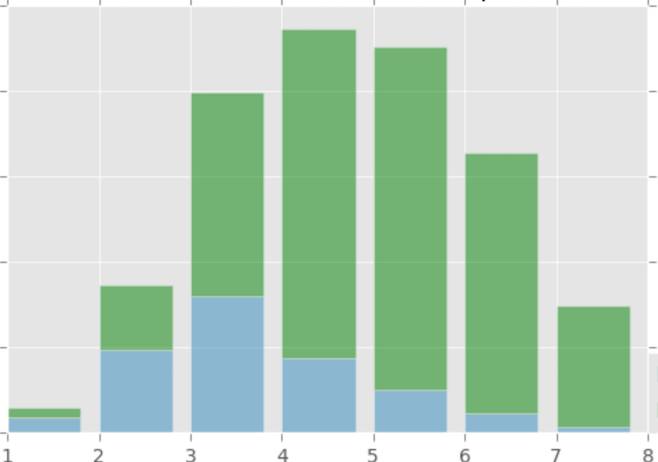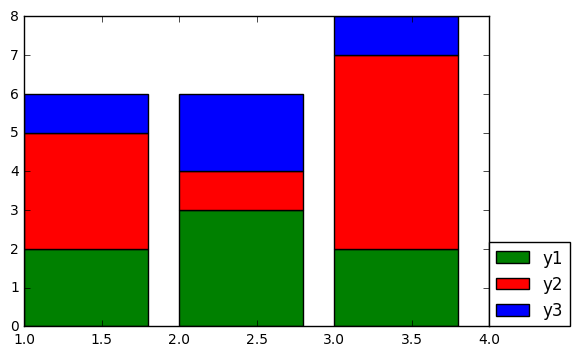#### 怎么用matplotlib.pyplot画出堆积柱状图？

统计/机器学习 数据可视化 Python    浏览次数：11611        分享

1姜金杰   2017-12-27 13:40

##### 1个回答
4

x = [1, 2, 3]
y1 = np.array([2, 3, 2])
y2 = np.array([3, 1, 5])
y3 = np.array([1, 2, 1])
plt.bar(x, y1, color='green', label='y1')
plt.bar(x, y2, bottom=y1, color='red', label='y2')
plt.bar(x, y3, bottom=y1+y2, color='blue', label='y3')
plt.legend(loc=[1, 0])
plt.show()SofaSofa数据科学社区DS面试题库 DS面经木子周   2017-12-28 13:42相关主题